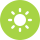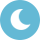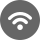# MTH 197: Linear Algebra

This is a first course in linear algebra. Topics include systems of linear equations, vector equations and matrix equations; matrix algebra, partitions and factorizations; determinants; matrix inverses and the Invertible Matrix Theorem; vector spaces and subspaces; linear independence, bases and dimension; null and column spaces, rank; linear transformations on vector spaces, kernel and range; injective, surjective and bijective mappings; isomorphism; eigenvalues and eigenspaces; diagonalization; inner product spaces, orthogonal matrices, Gram-Schmidt orthogonalization; least-squares approximation; and diagonalization of symmetric matrices.

### Class offerings by semester

Fall 2019 Winter 2020 SP/SU 2020Day Class (before 5:00pm)Evening Class (after 5:00pm)Online Class

Swipe left to see full chart
Description Hours
Credits  4
Lecture Hours 60
Clinical Hours 0
Lab Hours 0
Other Hours 0
Total Hours 60
Swipe left to see full chart